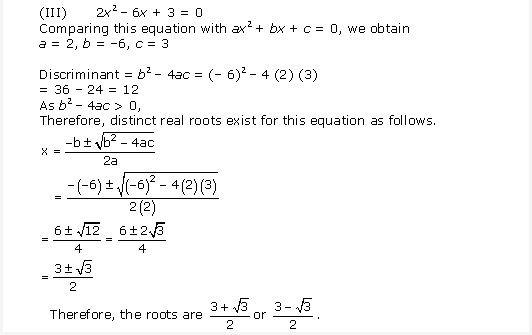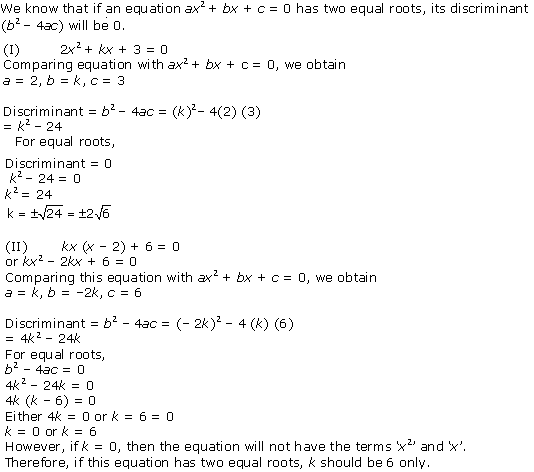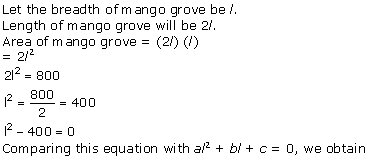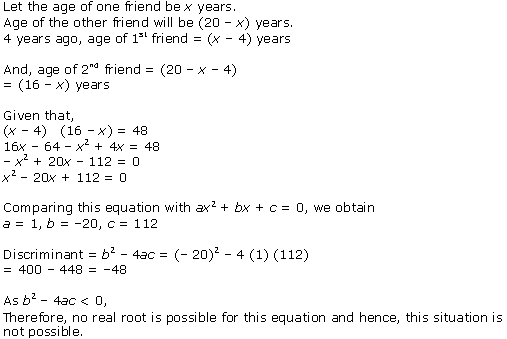# NCERT Solutions for Class 10 Maths Chapter 4 Quadratic Equations Ex 4.4

NCERT Solutions for Class 10 Maths Chapter 4 Quadratic Equations Ex 4.4 are part of . Here are we have given Chapter 4 Quadratic Equations Class 10 NCERT Solutions Ex 4.4.

 Board CBSE Textbook NCERT Class Class 10 Subject Maths Chapter Chapter 4 Chapter Name Quadratic Equations Exercise Ex 4.4 Number of Questions Solved 5 Category NCERT Solutions

## NCERT Solutions for Class 10 Maths Chapter 4 Quadratic Equations Ex 4.4

Page No: 91

Question 1. Find the nature of the roots of the following quadratic equations. If the real roots exist, find them;
(i) 2x2 – 3x + 5 = 0
(ii) 3x2 – 4√3x + 4 = 0
(iii) 2x2 – 6x + 3 = 0

Solution :Question 2. Find the values of k for each of the following quadratic equations, so that they have two equal roots.
(i) 2x2 + kx + 3 = 0
(ii) kx (x – 2) + 6 = 0

Solution :Question 3. Is it possible to design a rectangular mango grove whose length is twice its breadth, and the area is 800 m2? If so, find its length and breadth.

Solution :Question 4. Is the following situation possible? If so, determine their present ages. The sum of the ages of two friends is 20 years. Four years ago, the product of their ages in years was 48.

Solution :Question 5. Is it possible to design a rectangular park of perimeter 80 and area 400 m2? If so find its length and breadth.

Solution :We hope the NCERT Solutions for Class 10 Maths Chapter 4 Quadratic Equations Ex 4.4 help you. If you have any query regarding NCERT Solutions for Class 10 Maths Chapter 4 Quadratic Equations Ex 4.4, drop a comment below and we will get back to you at the earliest.